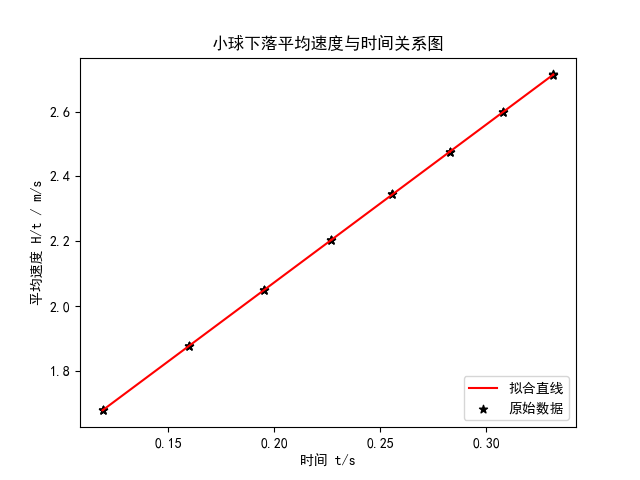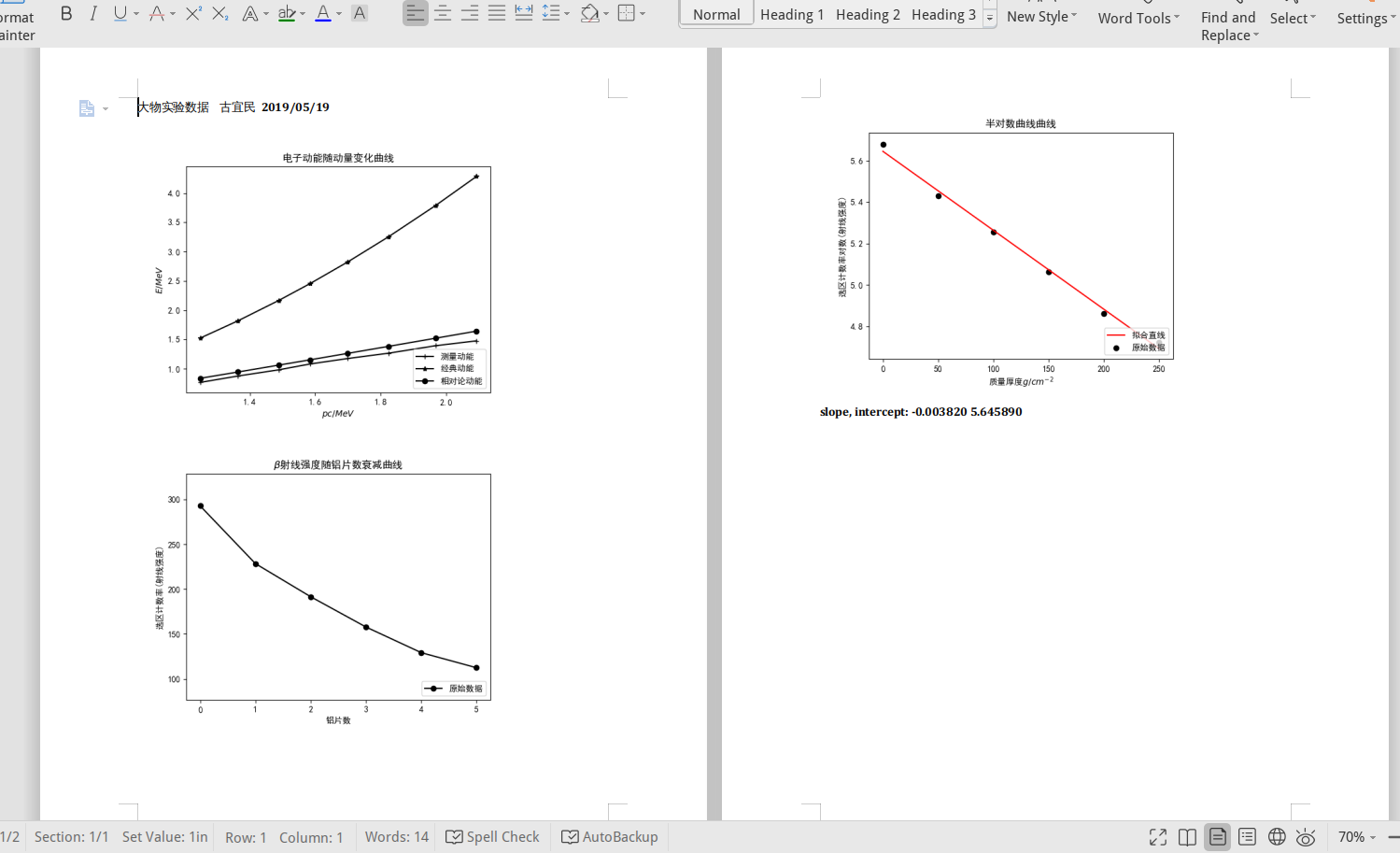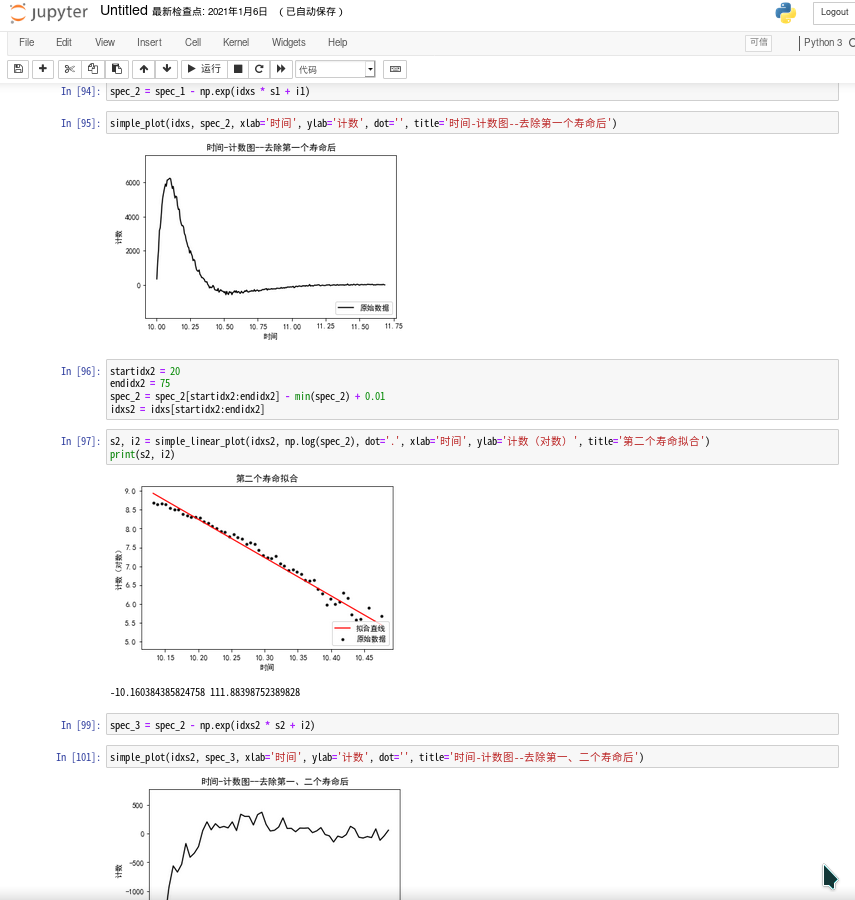## 开始

Python 做这些事情其实都不困难。画图用 Matplotlib 非常方便，也可以直接保存图片。数据计算自然是 NumPy。线性拟合的话，最初选择了 SciPy。输入输出还是比较基本，从文件读入。

``````#光电门间距 H cm
#数量级
e -2
90.0	90.0	90.0	80.0	80.0	80.0	70.0	70.0	70.0	60.0	60.0	60.0	50.0	50.0	50.0	40.0	40.0	40.0	30.0	30.0	30.0	20.0	20.0	20.0
#时间差 t ms
e -3
331.6	331.5	331.8	307.9	307.9	307.9	282.9	282.8	282.9	255.8	255.7	255.7	226.9	227.0	226.9	195.2	195.2	195.2	159.9	159.9	159.8	119.2	119.1	119.2
``````
``````#!/usr/bin/env python3
# -*- coding: utf-8 -*-
import numpy as np
import matplotlib.pyplot as plt
from scipy import stats
import math
#avoid font problem
plt.rcParams['font.sans-serif'] = ['SimHei']
plt.rcParams['axes.unicode_minus'] = False
data = []
#order of magnitude
oom = 0
fin = open('./data.txt', 'r')
if i == '#':
pass
elif i == 'e':
oom = int(i.split())
else:
data.append(np.array([float(x) * pow(10, oom) for x in i.split()]))
oom = 0
# print(data)
### main processing ###
l = len(data)
x = data
y0 = data
data.append(y0 / x)
y1 = data
slope, intercept, r_value, p_value, std_err = stats.linregress(x, y1)
# z = np.polyfit(x, y1, 1)
s_slope = slope * math.sqrt((r_value ** -2 - 1) / (l - 2))
s_intercept = s_slope * math.sqrt(np.mean(x ** 2))
print('linear regression:' )
print('slope:', slope)
print('intercept:', intercept)
print('r-value:', r_value)
print('p-value:', p_value)
print('std-err:', std_err)
print('r-squared:', r_value ** 2)
print('斜率标准差:', s_slope)
print('截距标准差:', s_intercept)
print('算得重力加速度:', 2 * slope)
#plot
plt.scatter(x, y1, marker='*', color='black', label='原始数据')
# plt.plot(x, y1, '--', color='green', label='光滑曲线')
plt.plot(x, intercept + slope * x, 'r', label='拟合直线')
plt.xlabel('时间 t/s')
plt.ylabel('平均速度 H/t / m/s')
plt.legend(loc=4)
plt.title('小球下落平均速度与时间关系图')
plt.savefig('pic.png')
plt.show()
``````## 两年之后

``````fin = open('./data.txt', 'r', encoding='utf-8')
fin.close()
``````

``````simple_plot(Momentum, Emeasure, show=0, issetrange=0, dot='+', lab='测量动能')
simple_plot(Momentum, Eclassic, show=0, issetrange=0, dot='*', lab='经典动能')
simple_plot(Momentum, Erela, dot='o', save='1.png', issetrange=0, xlab='\$pc/MeV\$', ylab='\$E/MeV\$', title='电子动能随动量变化曲线', lab='相对论动能')
``````

``````slope, intercept = simple_linear_plot(Al_Real, CntLn, xlab='质量厚度\$g/cm^{-2}\$', ylab='选区计数率对数(射线强度)', title='半对数曲线曲线', save='3.png')
print(-slope)
print(math.log(1e4) / (-slope))
print((math.log(Cnt) - 4 * math.log(10) - intercept) / slope)
``````

``````gendocx('gen.docx', '1.png', '2.png', '3.png', 'slope, intercept: %f %f' % (slope, intercept))
````````````#!/usr/bin/env python3
# -*- coding: utf-8 -*-

from physicsexp.mainfunc import *
from physicsexp.gendocx import *

fin = open('./data.txt', 'r', encoding='utf-8')
fin.close()

# data process

# calculated calibration values in class
a = 2.373e-3
b = -.0161
dEk = .20

c0 = 299792458.
MeV = 1e6 * electron

Emeasure = a * N + b + dEk
x0 = .10
R = (pos - x0) / 2
B = 640.01e-4
Momentum = 300 * B * R
Eclassic = ((Momentum * MeV)**2 / (2 * me * c0**2)) / MeV
Erela = np.array([math.sqrt((i * MeV)**2 + (me * c0**2)**2) - me * c0**2 for i in Momentum]) / MeV
print('pos\t', pos)
print('R\t', R*100)
print('pc\t', Momentum)
print('N\t', N)
print('Eclas\t', Eclassic)
print('Erela\t', Erela)
print('Emes\t', Emeasure)

simple_plot(Momentum, Emeasure, show=0, issetrange=0, dot='+', lab='测量动能')
simple_plot(Momentum, Eclassic, show=0, issetrange=0, dot='*', lab='经典动能')
simple_plot(Momentum, Erela, dot='o', save='1.png', issetrange=0,
xlab='\$pc/MeV\$', ylab='\$E/MeV\$', title='电子动能随动量变化曲线', lab='相对论动能')

Len = 150
Cnt = Cnt / Len
simple_plot(Al_num, Cnt, xlab='铝片数', ylab='选区计数率(射线强度)',
title='\$\\beta\$射线强度随铝片数衰减曲线', save='2.png')
CntLn = np.log(Cnt)
# d = 50 mg / cm^2
d = 50
Al_Real = Al_num * d
slope, intercept = simple_linear_plot(Al_Real, CntLn, xlab='质量厚度\$g/cm^{-2}\$', ylab='选区计数率对数(射线强度)',
title='半对数曲线曲线', save='3.png')
print(-slope)
print(math.log(1e4) / (-slope))
print((math.log(Cnt) - 4 * math.log(10) - intercept) / slope)

gendocx('gen.docx', '1.png', '2.png', '3.png', 'slope, intercept: %f %f' % (slope, intercept))
``````

## 迟到的 Jupyter Notebook